Zheng, S., Song, K., Zhao, J., and Dong, C. (2016). "Inverse estimation of effective moisture diffusivity in lumber during drying using genetic algorithms," BioRes. 11(4), 8226-8238.

#### Abstract

This article presents a methodology based on genetic algorithms (GA) optimization with a three-dimensional numerical solution to the diffusion model obtained by using the finite volume method (FVM) for determining the effective moisture diffusivity in lumber. The objective or error function between measured and simulated drying curves was obtained, and the effective moisture diffusivity parameters with greatest correspondence between measured and estimated values were obtained. As a result, a new equation for effective moisture diffusivity was proposed, which depends on lumber moisture content and drying temperature. Effective moisture diffusivities ranged from 1.120 × 10-9 to 1.277 × 10-8 m2/s. Finally, the proposed coefficients were validated by experiments. The drying kinetics were successfully simulated with the optimized effective moisture diffusivity model.

Inverse Estimation of Effective Moisture Diffusivity in Lumber during Drying Using Genetic Algorithms

Shaojiang Zheng,a,b Kuiyan Song,a,* Jingyao Zhao,a and Chunlei Dong b

This article presents a methodology based on genetic algorithms (GA) optimization with a three-dimensional numerical solution to the diffusion model obtained by using the finite volume method (FVM) for determining the effective moisture diffusivity in lumber. The objective or error function between measured and simulated drying curves was obtained, and the effective moisture diffusivity parameters with greatest correspondence between measured and estimated values were obtained. As a result, a new equation for effective moisture diffusivity was proposed, which depends on lumber moisture content and drying temperature. Effective moisture diffusivities ranged from 1.120 × 10-9 to 1.277 × 10-8 m2/s. Finally, the proposed coefficients were validated by experiments. The drying kinetics were successfully simulated with the optimized effective moisture diffusivity model.

Keywords: Effective moisture diffusivity; Genetic algorithms; Inverse problem; Optimization

Contact information: a: Material Science and Engineering College, Northeast Forestry University, Harbin 150040, China; b: Material Engineering College, Southwest Forestry University, Kunming 650224, China;

* Corresponding author: skuiyan@126.com

INTRODUCTION

Conventional drying is the most energy-intensive and time-consuming component of the lumber manufacturing process. Currently, the majority of the solid wood products available in the market are dried in batch driers in which the solid wood is exposed to hot air at controlled temperature and relative humidity. To optimize the process technology and reduce energy use, some physical experiments and theoretical models should be implemented. Compared with time-consuming experiments, mathematical models are useful in describing and understanding the process (Jia et al. 2015). Understanding moisture distributions is important because it enables the calculation of stresses that can damage the product during the drying process (Fu et al. 2013). It is therefore crucial to simulate the mass transfer of water within wood during the drying process.

Several mathematical models have been developed to simulate the mass transfer of water during green lumber drying. Although the empirical models (Zhan et al. 2007) and permeation models (Salin 2008) provide an approach in modeling, diffusion models (Dincer and Dost 1996; Silva et al. 2011, 2013; Zhao et al. 2016a) have been used frequently to describe the drying process. To consider liquid diffusion as the only mechanism of water mass transfer inside the lumber is a simplification of the model. However, drying kinetics are simulated with success through the accurate determination for effective moisture diffusivity. Using new mathematical approaches and modern computational facilities, the inverse method has become increasingly important for estimating coefficient values of moisture mass transfer in wood. For example, Liu et al. (2001) and Zhou et al. (2011) used a finite difference method to inversely determine the moisture diffusion coefficient. However, this numeral inverse problem is often described as ill-posed, and for this reason, it is sensitive to experimental measurement errors. Alternatively, Olek et al.(2005) optimized the inverse procedure to identify the diffusion coefficient. Eriksson et al. (2006) and Silva et al. (2011, 2013) successfully used this technique to determine effective moisture diffusivity in wood. Thus, the optimization of algorithms applied in the solving process may be crucial. The rationality, including computational accuracy and time of optimization algorithm, has never been verified, and the computer programming code is relatively complex. More importantly, the optimization algorithm is not easily extended.

Genetic algorithms (GA) inspired by Darwin’s theory of evolution have been used frequently for inverse and optimization problems in heat transfer (Louis et al. 2009; Zhao et al. 2016b). Because this algorithm has good generality, it may be a good method to determine the effective moisture diffusivity in lumber.

The main objectives of this study were to propose a three-dimensional numerical solution to the moisture diffusion equation using the finite volume method (FVM) in Cartesian coordinates and to use this solution together with genetic algorithms based on the inverse method to determine the effective moisture diffusivity. As a result, a new equation for the effective moisture diffusivity was proposed, which depends on moisture content and temperature. The proposed coefficient was validated experimentally.

EXPERIMENTAL

Materials and Methods

The experimental material was harvested from a larch plantation (Larix gmelinii Rupr.) in the Heilongjiang Province of China. Timbers were obtained from sapwood tangential cutting with the dimensions of 30 × 80 × 300 mm3, a basic density of 405 ± 9 kg/m3, and an initial moisture content of 112.4%, where moisture content was calculated as (green wt. – oven-dry wt.)/(oven-dry wt.). Prior to experimentation, the materials were carefully and slowly dried to moisture contents near 85%. To ensure an even profile of moisture content, conditioning and equalization treatments were performed during the drying process. All dried lumbers were sealed with two layers of plastic film to prevent water loss during the deposition.

Drying experiments were conducted in a DS-408 conditioning chamber constructed in Nanjing, China. The air velocity through the timber was approximately 2.0 m/s. The drying experiments were executed at different temperatures (T = 40, 50, 60 and 70 °C) and at the same equilibrium moisture content (about 9.6%). To ensure repeatability, the drying curve for each temperature was determined three times, and twelve curves were obtained. Every about 12 h during drying, specimens were removed quickly, and the moisture content was measured gravimetrically. When the average moisture content reached the equilibrium moisture content, the experimental drying runs were terminated.

Mathematical Model

A three-dimensional diffusion model was used to describe lumber drying. Through Fick’s second law, the diffusion equation in Cartesian coordinates (i.e., (x,y,z) in Fig. 1) was written as,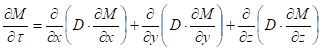(1)

where M is the moisture content in %,  is the time in s, and D is the effective moisture diffusivity in m2/s.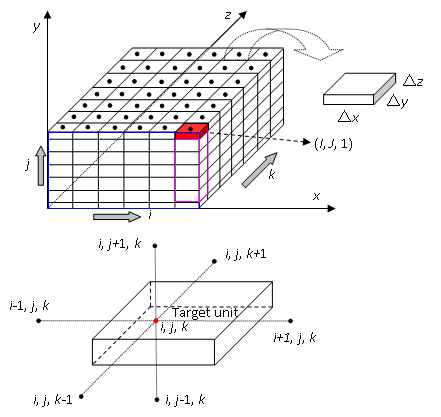Fig. 1. Types of control volumes in a three-dimensional domain

The following rational assumptions were established to solve Eq. 1, with the objective to apply the solution to lumber drying studies:

1. The convective mass transfer coefficient is constant over all surfaces of the timber during drying process.
2. Liquid diffusion is the only mechanism of mass transfer of water inside the lumber.
3. The effective moisture diffusivity can vary with moisture content and temperature during the drying process; the proportions in the three directions (radial, tangential and longitudinal) are DRDTDL = 1:1:ε. According to Olek et al. (2005), ε is equal to 6 to 7, and it is established by random number generation.
4. The external environment conditions, i.e., ambient temperature, humidity and velocity, do not change with the timber moisture content and temperature.
5. The timber structure is symmetric about the center.

The numerical solution was obtained by using the finite volume method expressed in explicit terms. Compared to implicit terms, an important feature of the explicit expression is it ease of writing and its use. One drawback of using this approach is the algorithm stability (Smith 1985). Through a preliminary study of the grid and time refinement, the space (x, y, z) and dimensions were set to 2 mm and 0.1 s, respectively, to ensure the stability of the algorithm. The control volume of the object was constructed using discrete I(ixJ(jyK(kz) units with the nodes located at the core of each unit (Fig. 1). The time domain was divided into n equal parts with a time step of. Various difference equations are defined below.

Internal control volume

Integrating Eq. 1 with respect to space (x, y, z) and time () yields the following expression,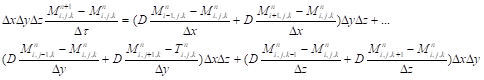(2)

where i = 2,3,…I – 1; j = 2,3,…J – 1; and k = 2,3,…K – 1.

Surface control volume

The control volume has a surface in contact with the external medium at its boundaries. Based on assumption (5) above, the structure is symmetric about its center. Thus, the surface control volume surrounded by the blue line in Fig. 1 is given. Other control volumes can be easily obtained using the same concept with reference to the following equation,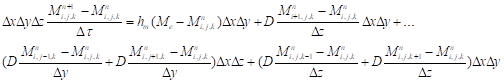(3)

where i = 2,3,…I – 1; j = 2,3,…J – 1; k = 2,3,…K – 1; hm is the convective mass transfer coefficient in kg/(m2∙s); and Me is environmental equilibrium moisture content in %.

Edge control volume

Compared with the surface control volume, the edge control volume includes a convective term instead of a diffusion term. The edge control volume surrounded by the pink line in Fig. 1 is given as,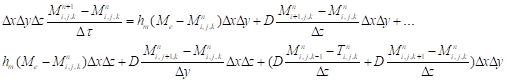(4)

where i = 2,3,…I – 1; j = 2,3,…J – 1; and k = 2,3,…K – 1.

Corner control volume

Based on the above difference equations, the corner control volume can be easily determined as,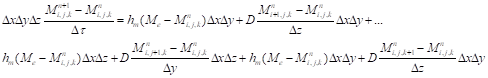(5)

where i = I; j = J; and k = K.

Average moisture content

Because the moisture content is determined for each control volume and time, the average value at time of can be calculated by,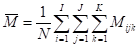(6)

where  is average moisture content in %; Mijk is moisture content of each control volume in %; and N is the total number of control volumes.

Effective moisture diffusivity

In solving all the difference equations, the diffusivity at the interfaces of the control volumes is calculated with the harmonic average criterion (Patankar 1980). Equation 7 defines the diffusivity at the interface between unit (i-1,j,k) and unit (i,j,k).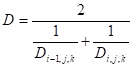(7)

According to Olek et al. (2005), the effective moisture diffusivity can be calculated using an exponential function with high accuracy. Thus, the function for effective moisture diffusivity can be expressed as follows, which is used to relate diffusivity and moisture content,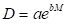(8)

where a and b are parameters to be determined by GA optimization (to be described in the next section). To relate the effective moisture diffusivity with temperature and moisture content, the expression proposed by Pan et al. (2007) is used (Eq. 9),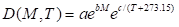(9)

where the equation coefficients ab and c are determined by curve fitting.

The convective mass transfer coefficient

In general, the convective mass transfer coefficient expresses moisture exchange capacity between the surface and environment, and is defined by Tremblay et al. (2000) as follows,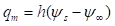(10)

where qm is moisture flux in kg/(m2∙s) and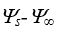represents the driving force behind moisture migration between the surface and the environment. The difference between surface moisture content and environmental equilibrium moisture content is considered as the driving force for this investigation; hence, the unit of the convective mass transfer coefficient is kg/(m2∙s).

Optimization Procedure

Unlike solving direct problems, the unknown parameter can be solved by an inverse approach based upon known experimental data. In this case, the effective moisture diffusivity and the convective mass transfer coefficient are regarded as unknown quantities. For estimation of such parameters, the average moisture content measurements of the lumber are known. Thus, the minimization of the difference between experimental and predicted moisture content values is desired. To quantify the similarity of the simulation results obtained from each value with the experimental moisture content, the Euclidean distance (Euclidea) was calculated in Eq. 11,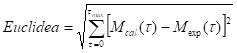(11)

where tmax is maximum time for analysis in s, Mcal is predicted moisture content in %, Mexp is experimental moisture content in %.

Thus, an optimization function based on the GA method finds the parameter that minimizes the Euclidean distance (i.e., least squares). The GA used in this investigation was previously published (Zhao et al. 2015b), where the algorithm method was used to determinate thermal conductivity. Details of the GA will be briefly reviewed as follows.

Genetic algorithm (GA)

This optimization algorithm is based on biological evolution (Michalewicz 1992). The GA consists of six basic steps: encoding, population initialization, fitness evaluation, selection, cross-over, and mutation. First, the unknown parameters are encoded as chromosomes of an individual. Values of these chromosomes are randomly assigned within specified ranges. By repeating the above random process, a certain number of individuals are generated. These individuals constitute the initial population. Second, by evaluating the fitness of the individuals in the initial population, the individuals of lower fitness are eliminated. Chromosomes of individuals with higher fitness are selected through the operations of cross-over and mutation; these selections generate a second-generation population. The population regeneration is repeated until a desired termination criterion is reached.

Fortunately, all of these basic algorithm steps can be run using GA toolboxes built into commercial software programs. For example, the GA Matlab® Toolbox (MathWorks 2014) was applied in the current study. Figure 2 shows a flowchart for estimating the effective moisture diffusivity parameters. However, finding the best GA parameter settings is not an easy task. GA parameters were set empirically with the aid of a training data set. The best settings were chosen and shown in Table 1. Each optimization approach was implemented in Matlab-2014b.

Table 1. Best Parameter Settings Used in Experiments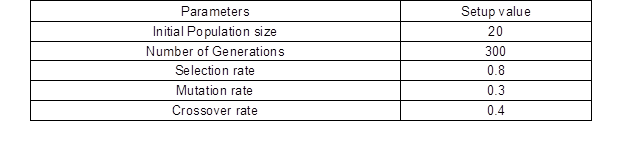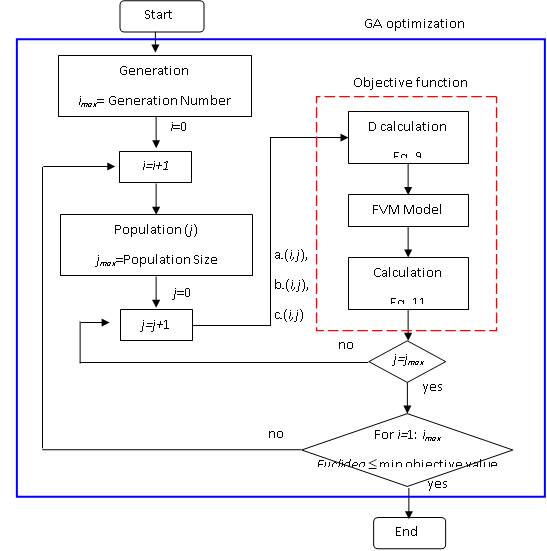Fig. 2. Process flow diagram to determine effective moisture diffusivity parameters

RESULTS AND DISCUSSION

According to Silva et al. (2013), the drying process, in many occasions, is considered isothermal because the thermal diffusivity is greater than the effective moisture diffusivity. This assumption was used in the present work.

Effective Moisture Diffusivity Estimation

To economize computing time, only a piece equivalent to 1/8 of the timber was modeled due to the inherent symmetry of the board (Fig. 3a). The numerical solution of Eq. 1 was performed through the finite volume method to obtain simulated results. Figure 3b shows moisture distribution in timber after 18 h of drying at 40 °C. The results from simulations were stable without volatility. The grid and time refinements used for the model simulation were enough to ensure stability of the algorithm. For each drying temperature, the effective moisture diffusivity was treated as a variable, which is a function of the local moisture content as given by Eq. 8. Thus, the process parameters determined from the optimization are summarized in Table 2. It was possible to conclude from this tabulated data that the proposed regression equation had a good statistical fit for the various drying temperatures. The multiple coefficient of correlations (R2) value of 0.9 to 1.0 is considered a good regression fit to the experimental data for estimating the diffusivity values obtained in this work. This observation is in agreement with the results of Silva et al. (2013), and indicated that Eq. 8 represented the relationship between moisture diffusivity and moisture content as rational.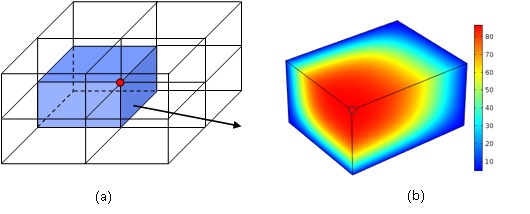Fig. 3. Symmetry sketches of timber and moisture distribution. (a) Highlighting a symmetrical piece; (b) moisture distribution after 18 h at 40 °C

Table 2. Results of the Optimization Processes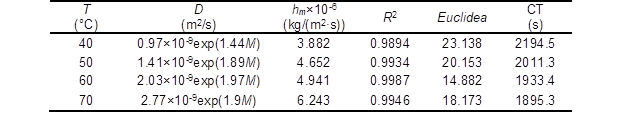CT, computing time

Figure 4 shows the results of the model simulations and experimental data of the drying kinetics at 40 °C. The difference between the experimental drying curves and those simulated using FVM with the GA optimization gradually converged with one another, i.e., good agreement was obtained after 300 optimization iterations.

Table 3 lists the effective moisture diffusivity for various drying temperature for moisture content (M) values ranging from 10 to 80%. The listed values are calculated using Eq. 8 with the coefficient values given in Table 2 for each T and M value.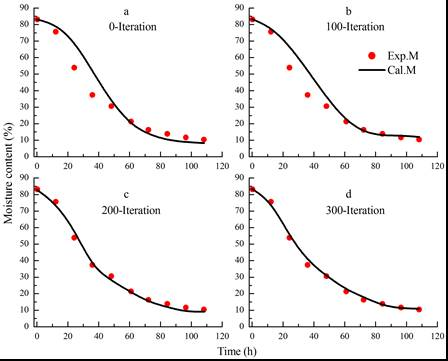Fig. 4. Model simulations and experimental data of the lumber drying kinetics at 40 °C

Table 3. Values of Effective Moisture Diffusivity (×10-9 m2/s) for Given Temperature and Moisture Content Values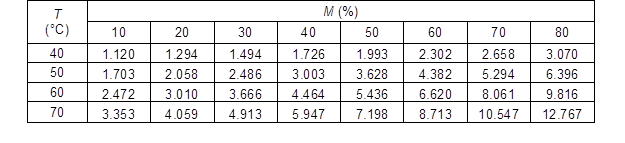The dependence of the effective moisture diffusivity (D) on both temperature (T) and moisture content (M) is obtained by curve fitting of Eq. 9 to the dataset of Table 3. The result is given by Eq. 12, which has an R2 value of 0.933: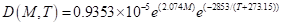(12)

The curve fitting of Table 3 data resulting in Eq. 12 is presented in Fig. 5. This figure reveals an expected result, i.e., effective moisture diffusivity increased with increasing temperature and with increasing moisture content. This trend of diffusivity was also noticed by Zhou et al. (2011) and Silva et al. (2013).

Effective Moisture Diffusivity Validation

To validate experimentally the proposed effective moisture diffusivity (Eq. 12), experimental drying curves were obtained for different temperatures (= 40 and 70 °C). The experimental values obtained during these tests were compared with those estimated by the FVM drying model using the proposed effective moisture diffusivity. Figure 6 shows that the estimated average moisture content from the model agreed well with the experimental data. The deviation between experimental and model calculated data may be attributed to wood properties and to a constant convective mass transfer coefficient. However, the drying curve calculated using the proposed effective moisture diffusivity was adequate to simulate the drying process with high accuracy, as shown in Fig. 6. Therefore, no further optimization was done when considering the high computing time (CT) with GA optimization and the satisfactory simulation results.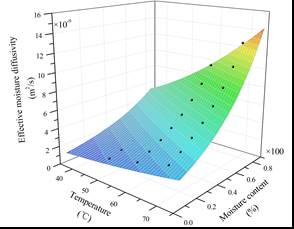Fig. 5. The effective moisture diffusivity as a function of the temperature and the moisture content within the lumber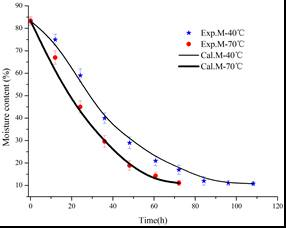Fig. 6. The comparison of model simulations and experiments of the lumber drying kinetics

Discussion of Moisture Transfer in Wood

Water migrates in the form of liquid and vapor from the interior to exterior of wood during drying. Liquid migration occurs through permeation above the fiber saturation point (FSP) and diffusion below it. If all of the above conditions are considered when describing the complete drying process, the model becomes quite complex and is difficult to solve numerically. Furthermore, it is difficult to obtain accurate values of relevant parameters, i.e., liquid permeability and mass diffusivity. Obviously, the consideration of liquid diffusion as the only mechanism of water transport inside the lumber is a simplification inherent in the model. However, the model simulation of the drying kinetics had high degree of accuracy when compared with actual data. This high accuracy was archived through the FVM drying model and optimization method based on GA. Inspection of the obtained results suggested that the liquid diffusion model with these proposed parameters adequately describes the lumber-drying process. In this study, three principal reasons were considered for using the diffusion model to describe the drying process.

1. The fiber saturation point (FSP) is considered the boundary between free water and bound water within the wood, where free-water transfer is mainly via permeation or pore diffusion and bound-water transfer is via diffusion or surface diffusion (Bedane et al. 2016). However, this characterization is an idealized condition. In practice, diffusion occurs throughout the entire drying process, especially for tree species with low permeability.
2. During isothermal drying, the pressure gradient according to Darcy’s law reduces to a moisture-content gradient, and the mathematical expression becomes equivalent to Fick’s second law (Zhao et al. 2016a). Furthermore, the traditional permeability coefficients take the form of pseudo-diffusion coefficients (Katekawa and Silva 2006).
3. Through optimization with a numerical solution, effective moisture diffusivity can be adjusted to reduce the differences between simulation and experimental values. Thus, the simulation of lumber drying kinetics was considered a success; the diffusion model can be used to describe the complete drying process.

CONCLUSIONS

1. In this study, a three-dimensional numerical solution to the diffusion equation with variable effective moisture diffusivity and constant convective mass transfer coefficient, obtained via the finite volume method, is proposed to describe the drying of wood.
2. The proposed coefficient was validated using experimental drying curves obtained for various drying temperatures. The drying curves calculated using the proposed effective moisture diffusivity showed good agreement with the experimental data, which was dependent on moisture content and temperature.
3. In contrast to traditional techniques, the proposed methodology was used to effectively and economically determine effective moisture diffusivity. The proposed effective moisture diffusivity can be used for simulating drying kinetics.

ACKNOWLEDGMENTS

The authors are grateful for the support of the Special Scientific Research Fund of Foundation of Yunnan Provincial Education Department (No.2015Y291).

REFERENCES CITED

Bedane, A. H., Eić, M., Farmahini-Farahani, M., and Xiao, H. (2016). “Theoretical modeling of water vapor transport in cellulose-based materials,” Cellulose 1-16.

DOI10.1007/s10570-016-0917-y

Dincer, I., and Dost, S. (1996). “A modeling study for moisture diffusivities and moisture transfer coefficients in drying of solid objects,” Int. J. Energ. Res. 20(6), 531-539. DOI: 10.1002/(SICI)1099-114X

Fu, Z. Y., Cai, Y. C., Zhao, J. Y., and Huan, S. Q. (2013). “The effect of shrinkage anisotropy on tangential rheological properties of Asian white birch disks,” BioResources 8(4), 5235-5243. DOI: 10.15376/biores.8.4.5235-5243

Jia, X. R., Zhao, J. Y., and Cai, Y. C. (2015). “Radio frequency vacuum drying of timber: Mathematical model and numerical analysis,” BioResources 10(3), 5440-5459. DOI: 10.15376/biores.10.3.5440-5459

Eriksson, J., Johansson, H., and Danvind, J. (2006). “Numerical determination of diffusion coefficients in wood using data from CT-scanning,” Wood Fiber Sci. 38(2), 334-344.

Katekawa, M. E., and Silva, M. A. (2006). “A review of drying models including shrinkage effects,” Dry. Technol. 24(1), 5-20. DOI: 10.1080/07373930500538519

Liu, J. Y., Simpson, W. T., and Verrill, S. P. (2001). “An inverse moisture diffusion algorithm for the determination of diffusion coefficient,” Dry. Technol. 19(8), 1555-1568. DOI: 10.1081/DRT-100107259

Louis, G., Maxime, T. G., and François, M. P. (2009). “Review of utilization of genetic algorithms in heat transfer problems,” Int. J. Heat Mass Tran. 52(9), 2169-2188. DOI: 10.1016/j.ijheatmasstransfer.2008.11.015

MathWorks (2014). Matlab User Guide, The MathWorks, Inc., Natick, MA, (http://www.mathworks.com).

Michalewicz, Z. (1992). Genetic Algorithms + Data Structures = Evolution Programs, Springer-Verlag, New York, NY.

Olek, W., Perre, P., and Weres, J. (2005). “Inverse analysis of the transient bound water diffusion in wood,” Holzforschung 59(59), 38-45. DOI: 10.1515/HF.2005.007

Pan, K. Y., Wang, X. Z., and Liu, X. D. (2007). Modern Drying Technology, 2nd Ed., Chemical Industry Press, Beijing, China (in Chinese).

Patankar, S. V. (1980). Numerical Heat Transfer and Fluid Flow, Hemisphere Publishing Corp., New York, NY.

Salin, J. G. (2008). “Drying of liquid water in wood as influenced by the capillary fiber network,” Dry. Technol. 26(5), 560-567. DOI: 10.1080/07373930801944747

Silva, W. P., Silva, L. D., Farias, V. S. O., Silva, C. M. D. P. S., and Ataide, J. S. P. (2013). “Three-dimensional numerical analysis of water transfer in wood: Determination of an expression for the effective mass diffusivity,” Wood Sci. Technol. 47(5), 897-912. DOI: 10.1007/s00226-013-0544-9

Silva, W. P., Silva, L. D., Silva, C. M. D. P. S., and Nascimento, P. L. (2011). “Optimization and simulation of drying processes using diffusion models: Application to wood drying using forced air at low temperature,” Wood Sci. Technol. 45(4), 787-800. DOI: 10.1007/s00226-010-0391-x

Smith, G. D. (1985). Numerical Solution of Partial Differential Equations, 3rd Ed., Oxford University Press, Oxford, UK.

Tremblay, C., Cloutier, A., and Fortin, Y. (2000). “Experimental determination of the convective heat and mass transfer coefficients for wood drying,” Wood Sci. Technol. 34(3), 253-276. DOI: 10.1007/s002260000045

Zhan, J. F., Gu, J. Y., and Cai, Y. C. (2007). “Analysis of moisture diffusivity of larch timber during convective drying condition by using Crank’s method and Dincer’s method,” J. Forest Res. 18(3), 199-202. DOI: 10.1007/s11676-007-0040-x

Zhao, J. Y., Fu, Z. Y., Jia, X. R., and Cai, Y. C. (2016a). “Modeling conventional drying of wood: Inclusion of a moving evaporation interface,” Dry. Technol. 34(5), 530-538. DOI: 10.1080/07373937.2015.1060999

Zhao, J. Y., Fu, Z. Y., Jia, X. R., and Cai, Y. C. (2016b). “Inverse determination of thermal conductivity in lumber based on genetic algorithms,” Holzforschung 70(3), 235-241. DOI: 10.1515/hf-2015-0019

Zhou, Q. F., Cai, Y. C., Xu, Y., and Zhang, X. L. (2011). “Determination of moisture diffusion coefficient of larch board with finite difference method,” BioResources 6(2), 1196-1203. DOI: 10.15376/biores.6.2.1196-1203

Article submitted: May 19, 2016; Peer review completed: July 18, 2016; Revised version received and accepted: July 26, 2016; Published: August 10, 2016.

DOI: 10.15376/biores.11.4.8226-8238